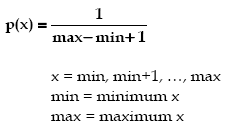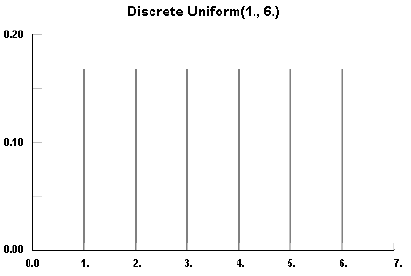AnyLogic

# uniform_discrThe Discrete Uniform distribution is a discrete distribution bounded on [min, max] with constant probability at every value on or between the bounds. Sometimes called the discrete rectangular distribution, it arises when an event can have a finite and equally probable number of outcomes.

### Sample### uniform_discr(int max)

Description
Generates a sample of the Discrete Uniform distribution in the interval [0, max], both 0 and max included! Is equivalent to uniform_discr(0, max). For more details see uniform_discr(int, int).
Parameters
Name Type Description
max int the maximum x value
Result
Type Description
double the generated sample

### uniform_discr(int min, int max)

Description
Generates a sample of the Discrete Uniform distribution on the interval [min, max], both min and max included!
Parameters
 Name Type Description min int the minimum x value max int the maximum x value
Result
Type Description
double the generated sample

### uniform_discr(int min, int max, java.util.Random r)

Description
Generates a sample of the Discrete Uniform distribution on the interval [min, max] using the specified random number generator, both min and max included! For more details see uniform_discr(int, int).
Parameters
Parameters
Name Type Description
min int the minimum x value
max int the maximum x value
r java.util.Random the random number generator
Result
Type Description
double the generated sample